38×12×0.838×12×1.038×12×1.238×20×0.638×20×0.850×0.550×1.550×12×1.250×12×1.050×15×1.050×15×1.250×15×1.550×19×0.4550×19×0.550×20×0.650×20×0.750×40×0.750×40×0.650×40×0.850×45×0.650×45×0.750×45×0.850×50×0.750×50×0.6100×45×0.860×27×1.5100×50×0.675×50×0.675×40×0.650×50×0.875×50×1.560×27×0.7150×40×1.0100×40×1.0100×50×1.275×0.675×50×1.2150×40×0.8100×40×0.875×45×0.860×27×1.2150×50×1.5100×42×0.875×40×1.5150×40×0.7100×40×0.775×45×0.760×27×1.075×42×0.8100×40×0.675×45×0.660×27×0.675×35×0.5150×50×1.0100×50×1.075×50×1.075×40×1.060×27×0.575×40×1.2150×50×0.8100×50×0.875×50×0.875×40×0.8100×40×1.2100×45×0.6100×45×0.7150×50×0.7100×50×0.775×50×0.775×40×0.7150×40×1.538×12×175×35×0.6100×50×1150×50×150×35×0.675×50×150×15×1100×40×130×20×0.875×40×1150×40×137×20×0.850×0.650×25×0.850×40×150×50×1100×0.5100×0.6100×0.8100×1100×35×0.7100×40×0.55100×47×0.7100×50×0.55120×40×1120×50×1150×40×1.2150×45×0.8150×47×0.8150×50×1.222×37×0.838×12×0.950×19×0.650×20×0.550×50×0.55600×600×1460×24×1.260×27×0.5560×27×175×0.575×0.875×40×0.5575×47×0.675×50×0.55C50×50×0.6C75×50×0.6U50×40×0.6U75×40×0.6100×26×0.8100×30×20×0.8100×35×0.6100×35×0.8100×37×0.8100×37×1100×42×1100×47×0.8103×50×25×0.810×38×0.510×55×0.610×55×0.811×55×0.6146×42×0.8146×42×1150×26×1150×37×1150×45×1150×47×115×20×25×0.520×0.520×10×0.820×20×200.420×20×30×0.520×20×34×0.520×20×34×0.620×22×120×25×2020×28×30×0.520×28×30×0.620×38×0.822×38×0.522×55×0.522×55×0.625×20×30×0.525×39×0.825×39×1.027×20×0.427×20×0.528×0.628×27×0.53000×40×303000×55×223000×65×3530×20×0.630×20×130×20×20×0.530×23×0.630×28×20×0.630×30×0.537×0.637×20×0.637×20×1.037×22×0.637×22×0.838×0.838×0.938×138×1.238×10×10×1538×11×0.538×12×0.638×20×0.750×0.4750×0.6×300050×150×1.250×12×150×25×150×35×0.750×40×0.5550×47×0.650×47×0.750×47×0.850×70×0.5550×（45/47）×0.655×11×0.8600×600×0.760×0.660×1.260×25×162×0.6×240073.5×45×0.673×35×0.67550×0.875×0.5575×175×10×0.675×26×0.875×30×20×0.875×35×0.4575×35×0.775×35×0.875×37×0.875×45×0.4575×45×0.575×47×0.775×47×0.875×（45/47）×0.678×50×25×0.898.5×45×0.6B60×27×0.6C100×0.7C100×45×0.8C100×50×0.6C100×50×0.7C100×50×0.8C150×50×0.8C50×0.6C50×50×0.8C50×50×07C75×0.6C75×50×0.8CB29×25×0.8CB38×12×1CB50×19×O.5CB50×20×0.6CB60×27×0.6CH100×42×0.8CH75×42×0.8CS50×15×1.2CS60×24×1.2CS60×27×1.2DF50××0.5DF60×0.6DM38×1.0DM50×1.2DM60×1.2DU30×20×20×0.5DU30×28×20×0.6DV37×20×0.8E100×30×20×0.8E75×30×20×0.8F30×50×20×0.5F30×60×20×0.5J103×50×25×0.8J78×50×25×0.8LDC50×19×0.45LDC60×27×0.5LDC60×27×1LDU50×15×1LQC75×45×0.6LQU75×35×0.6S50×15×1.2U100×0.7U100×35×0.7U100×40×0.6U100×40×0.7U100×40×0.8U150×40×0.8U20×27×20×0.4U30×20×27×0.6U30×20×30×0.5U50×0.6U50×35×0.6U50×35×0.7U75×0.6U75×40×0.8V37×20×0.6V37×20×0.8Z75×50×0.6×3000ZDV20×37×1ZDV20×38×0.8

C型U型V型CH型DM型E型J型L型T型Z型F型DU型H型

38系列50系列E型龙骨J型龙骨LLD-CO

2020201920182017

E型龙骨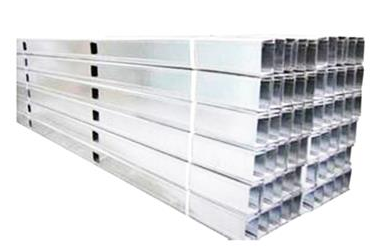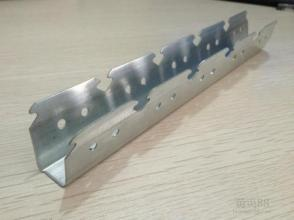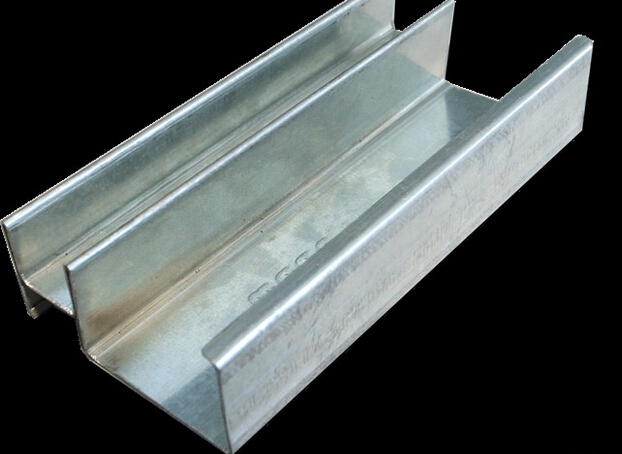m

13%
2020/10/22

m

13%
2020/09/25
/

E型龙骨m

13%
2020/10/22

m

13%
2020/09/25
/

CH龙骨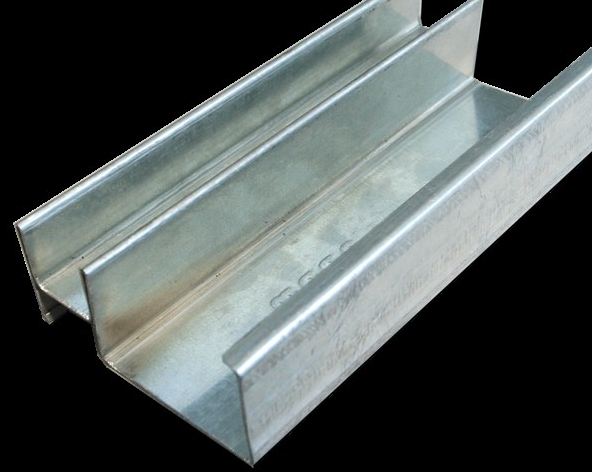m

13%
2020/10/22

m

13%
2020/09/25
/

CH龙骨m

13%
2020/10/22

m

13%
2020/09/25
/

J型龙骨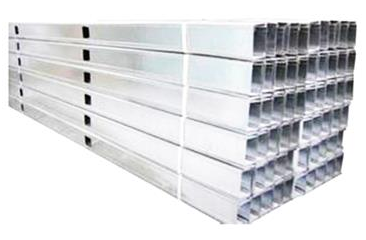m

13%
2020/10/22

J型龙骨m

13%
2020/10/22

QC-竖龙骨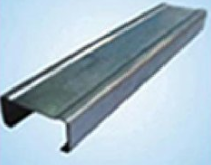m

13%
2020/10/22

QC-竖龙骨m

13%
2020/10/22

QU-横龙骨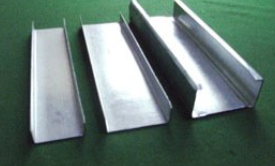m

13%
2020/10/22

QU-横龙骨m

13%
2020/10/22

QC-竖龙骨m

13%
2020/10/22

QC-竖龙骨m

13%
2020/10/22

QC-竖龙骨m

13%
2020/10/22

QC-竖龙骨m

13%
2020/10/22

QU-横龙骨m

13%
2020/10/22

QU-横龙骨m

13%
2020/10/22

QU-横龙骨m

13%
2020/10/22

QU-横龙骨m

13%
2020/10/22

QC-竖龙骨m

13%
2020/10/22

QC-竖龙骨m

13%
2020/10/22

QC-竖龙骨m

13%
2020/10/22

QU-横龙骨m

13%
2020/10/22

QU-横龙骨m

13%
2020/10/22

QU-横龙骨m

13%
2020/10/22

QC-竖龙骨m

13%
2020/10/22

QC-竖龙骨m

13%
2020/10/22

QU-横龙骨m

13%
2020/10/22

QU-横龙骨m

13%
2020/10/22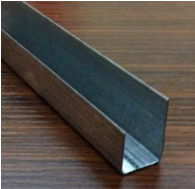m

13%
2020/10/22m

13%
2020/10/22m

13%
2020/10/22m

13%
2020/10/22

C2开启式边骨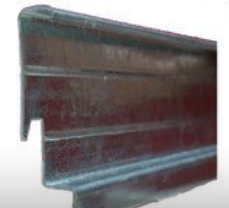m

13%
2020/10/22

C2开启式龙骨m

13%
2020/10/22

C2开启式龙骨m

13%
2020/10/22

C2开启式龙骨m

13%
2020/10/22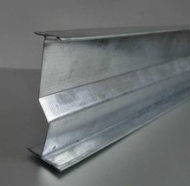m

13%
2020/10/22m

13%
2020/10/22m

13%
2020/10/22m

13%
2020/10/22

• 1
• 2
• 3
• 4
• 5
• 6
• 19订阅中心广联达大数据